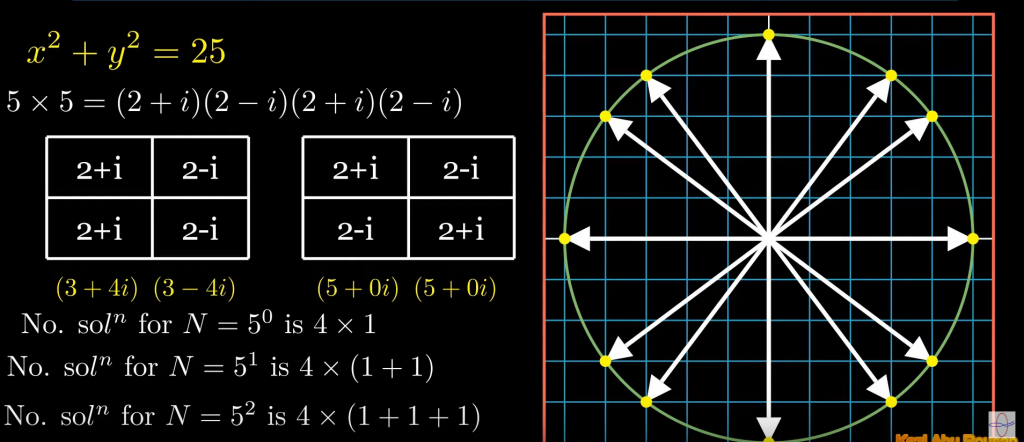How Cheenta works to ensure student success?
Explore the Back-Story

# Lattice points on a circle - No. of solution of x^2+y^2 = NAuthor: Kazi Abu Rousan

There are some problems in number theory which are very important not only because they came in exams but also they hide much richer intuition inside them. Today, we will be seeing one of such problems.

###### Sources:
• B.Stat. (Hons.) and B.Math. (Hons.) I.S.I Admission Test 2012 problem-2.
• B.Stat. (Hons.) and B.Math. (Hons.) I.S.I Admission Test 2015 problem-12.
• The classical Gauss circle problem.
###### Problem:

The basic structure of the problem we are discussing is:

Find the number of solution of the equation $x^2+y^2 = N$ where $N \in \mathbb{N}$.

So, How to solve problems like this?, Here I will give you a formula to exactly find the number of solution for any general integer $N$.

###### Solution

The main idea behind these kind of problem is to use Fermat's Two-Square Theorem. So, what does the theorem says?

It says:

If any prime number $N$ is of the form $4k+1$, then $N$ can be written as $x^2+y^2$ for some $x,y \in \mathbb{I}$. This means if $N$ is of the form $4k+3$, then $x^2+y^2=N$ doesn't have any solution.

This gives us our answer if $N$ is a prime number. So, if the problem is to find the number of solution of $x^2+y^2 = 2003$ or maybe $3, 7, \cdots , 23, \cdots$, i.e., any prime with the remainder of $3$ when divided by 4, then we directly know that the number of solution is zero.

So, we have a method to solve these type of problems for prime $N$. But what about non-prime numbers?

For the case of non-prime numbers, I will just give you the formula and will show you how to use that. If you want to know how we got the formula or the details of the formula, you can just watch my lecture.

The formula to calculate the number of solution for $N = p_0^{a_0} p_1^{a_1} \cdots p_{n-1}^{a_{n-1}}$

$$\text{ No of soln } = 4\cdot \Pi_{i=0}^{n-1}\Big(\sum_{j=0}^{a_i} \chi(p_i ^{j}) \Big)$$

or in simple terms,

$$\text{ No of soln } = 4\cdot (\chi(p_0^0)+\chi(p_0^1)+\cdots + \chi(p_0^{a_0}))\cdot ( \chi(p_1^0)+\chi(p_1^1)+\cdots + \chi(p_1^{a_1}) ) \cdots$$

Where $\chi$ is a number theoretical function defined as,

$\chi(x) = -1$ if $x = 4k+3$

$\chi(x) = 0$ if $x = 2^k$

$\chi(x) = 1$ if $x = 4k+1$.

and also $\chi(ab) = \chi(a) \times \chi(b)$

Let's see an example,

Suppose $N = 2250$. Now, $2250 = 2\times 3^2 \times 5^3$.

So, the number of solutions is, $n = 4\times (\chi(2^0)+\chi(2^1))\times (\chi(3^0)+\chi(3^1) + \chi(3^2)) \times (\chi(5^0)+\chi(5^1)+\chi(5^2)+\chi(5^3))$.

Now, from definition, $\chi(1) = 1$, $\chi(2) = 0$, $\chi(3) = -1$ and $\chi(5) = 1$. And also $\chi(x^n) = \chi(x)^n$, hence

$$n = 4\times (1+0) \times (1-1+1) \times (1+1+1+1) = 16$$.

So, I hope it is now clear on how to use that particular formula.

This is all for today. I hope you have learnt something new.

Author: Kazi Abu Rousan

There are some problems in number theory which are very important not only because they came in exams but also they hide much richer intuition inside them. Today, we will be seeing one of such problems.

###### Sources:
• B.Stat. (Hons.) and B.Math. (Hons.) I.S.I Admission Test 2012 problem-2.
• B.Stat. (Hons.) and B.Math. (Hons.) I.S.I Admission Test 2015 problem-12.
• The classical Gauss circle problem.
###### Problem:

The basic structure of the problem we are discussing is:

Find the number of solution of the equation $x^2+y^2 = N$ where $N \in \mathbb{N}$.

So, How to solve problems like this?, Here I will give you a formula to exactly find the number of solution for any general integer $N$.

###### Solution

The main idea behind these kind of problem is to use Fermat's Two-Square Theorem. So, what does the theorem says?

It says:

If any prime number $N$ is of the form $4k+1$, then $N$ can be written as $x^2+y^2$ for some $x,y \in \mathbb{I}$. This means if $N$ is of the form $4k+3$, then $x^2+y^2=N$ doesn't have any solution.

This gives us our answer if $N$ is a prime number. So, if the problem is to find the number of solution of $x^2+y^2 = 2003$ or maybe $3, 7, \cdots , 23, \cdots$, i.e., any prime with the remainder of $3$ when divided by 4, then we directly know that the number of solution is zero.

So, we have a method to solve these type of problems for prime $N$. But what about non-prime numbers?

For the case of non-prime numbers, I will just give you the formula and will show you how to use that. If you want to know how we got the formula or the details of the formula, you can just watch my lecture.

The formula to calculate the number of solution for $N = p_0^{a_0} p_1^{a_1} \cdots p_{n-1}^{a_{n-1}}$

$$\text{ No of soln } = 4\cdot \Pi_{i=0}^{n-1}\Big(\sum_{j=0}^{a_i} \chi(p_i ^{j}) \Big)$$

or in simple terms,

$$\text{ No of soln } = 4\cdot (\chi(p_0^0)+\chi(p_0^1)+\cdots + \chi(p_0^{a_0}))\cdot ( \chi(p_1^0)+\chi(p_1^1)+\cdots + \chi(p_1^{a_1}) ) \cdots$$

Where $\chi$ is a number theoretical function defined as,

$\chi(x) = -1$ if $x = 4k+3$

$\chi(x) = 0$ if $x = 2^k$

$\chi(x) = 1$ if $x = 4k+1$.

and also $\chi(ab) = \chi(a) \times \chi(b)$

Let's see an example,

Suppose $N = 2250$. Now, $2250 = 2\times 3^2 \times 5^3$.

So, the number of solutions is, $n = 4\times (\chi(2^0)+\chi(2^1))\times (\chi(3^0)+\chi(3^1) + \chi(3^2)) \times (\chi(5^0)+\chi(5^1)+\chi(5^2)+\chi(5^3))$.

Now, from definition, $\chi(1) = 1$, $\chi(2) = 0$, $\chi(3) = -1$ and $\chi(5) = 1$. And also $\chi(x^n) = \chi(x)^n$, hence

$$n = 4\times (1+0) \times (1-1+1) \times (1+1+1+1) = 16$$.

So, I hope it is now clear on how to use that particular formula.

This is all for today. I hope you have learnt something new.

This site uses Akismet to reduce spam. Learn how your comment data is processed.

### Knowledge Partner